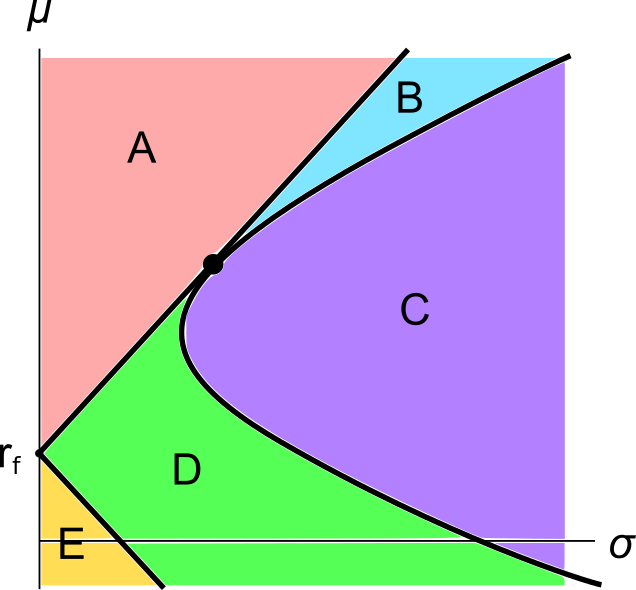# Fight Finance

#### CoursesTagsRandomAllRecentScores

A stock is expected to pay the following dividends:

 Cash Flows of a Stock Time (yrs) 0 1 2 3 4 ... Dividend ($) 2 2 2 10 3 ... After year 4, the dividend will grow in perpetuity at 4% pa. The required return on the stock is 10% pa. Both the growth rate and required return are given as effective annual rates. What will be the price of the stock in 5 years (t = 5), just after the dividend at that time has been paid? A firm wishes to raise$10 million now. They will issue 6% pa semi-annual coupon bonds that will mature in 3 years and have a face value of $100 each. Bond yields are 5% pa, given as an APR compounding every 6 months, and the yield curve is flat. How many bonds should the firm issue? An investor bought a 20 year 5% pa fixed coupon government bond priced at par. The face value is$100. Coupons are paid semi-annually and the next one is in 6 months.

Six months later, just after the coupon at that time was paid, yields suddenly and unexpectedly rose to 5.5% pa. Note that all yields above are given as APR's compounding semi-annually.

What was the bond investors' historical total return over that first 6 month period, given as an effective semi-annual rate?

What is the correlation of a variable X with itself?

The corr(X, X) or $\rho_{X,X}$ equals:

Which of the following interest rate quotes is NOT equivalent to a 10% effective annual rate of return? Assume that each year has 12 months, each month has 30 days, each day has 24 hours, each hour has 60 minutes and each minute has 60 seconds. APR stands for Annualised Percentage Rate.

A graph of assets’ expected returns $(\mu)$ versus standard deviations $(\sigma)$ is given in the below diagram.

Each letter corresponds to a separate coloured area. The portfolios at the boundary of the areas, on the black lines, are excluded from each area. Assume that all assets represented in this graph are fairly priced, and that all risky assets can be short-sold.

Which of the following statements about this graph and Markowitz portfolio theory is NOT correct?A European call option should only be exercised if:

A 12 month European-style call option with a strike price of $11 is written on a dividend paying stock currently trading at$10. The dividend is paid annually and the next dividend is expected to be \$0.40, paid in 9 months. The risk-free interest rate is 5% pa continuously compounded and the standard deviation of the stock’s continuously compounded returns is 30 percentage points pa. The stock's continuously compounded returns are normally distributed. Using the Black-Scholes-Merton option valuation model, determine which of the following statements is NOT correct.

Question 854  speculation motive for keeping money, no explanation

What is the speculation motive for keeping money? The speculation motive encourages people to keep money available:

A stock has an expected return of 10% pa and you're 90% sure that over the next year, the return will be between -15% and 35%. The stock's returns are normally distributed. Note that the Z-statistic corresponding to a one-tail:

• 90% normal probability density function is 1.282.
• 95% normal probability density function is 1.645.
• 97.5% normal probability density function is 1.960.

What is the stock’s standard deviation of returns in percentage points per annum (pp pa)?

Copyright © 2014 Keith Woodward